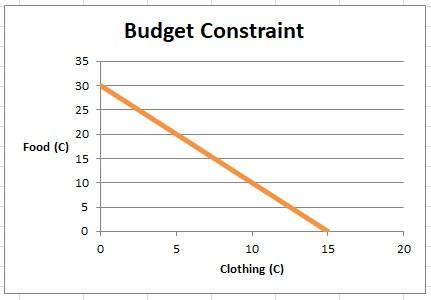# Suppose you have $120 to spend on food and clothing. The price of food is$4 and the price of...

Suppose you have $120 to spend on food and clothing. The price of food is$4 and the price of clothing is $8. a. Graph your budget constraint; calculate the slope of it. b. Briefly explain why your optimal choice is the point at which your budget constraint is tangent to an indifference curve. ## Budget Constraint: The combinations of goods and services that a consumer can purchase with the income at his or her disposal based on the current prices of those goods and services can be expressed in form of a budget constraint. The budget constraint shows that because the total cost of the goods and services in the consumer's basket cannot exceed the income, to consume one more unit of one good or service, some units of another good or a service has to be given up. ## Answer and Explanation: 1 a. Graph your budget constraint; calculate the slope of it Let food be F and clothing C such that the budget constraint is expressed as follows: {eq}$4F + $8C =$120 {/eq}

{eq}F = \$30 - 2C {/eq}. The graph of this budget constraint is given as follows:The slope of the equation can be computed as {eq}\frac{\Delta F}{\Delta C} = -2 {/eq}

b. Briefly explain why your optimal choice is the point at which your budget constraint is tangent to an indifference curve.

When maximizing utility, the consumer is constrained by the budget constraint. For any bundle that the consumer chooses to derive utility from, their total expenditure cannot exceed the budget. Since the consumer is rational, they will prefer to consume at a higher indifference curve. Utility is only maximized when the budget constraint curve and indifference curve and as the consumer chooses a higher indifference curve, the highest indifference curve is the one that is tangent to the indifference curve. It is the point when the slope of the budget constraint is equal to the slope of the indifference curve. It is the point where marginal rate of substitution of one good for another equals the ratio of their prices. That is, {eq}MRS_{xy} = \frac{P_X}{P_y} {/eq}